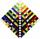# Sequence

Write the first 7 members of an arithmetic sequence: a1=-3, d=6.

Result

a1 =  -3
a2 =  3
a3 =  9
a4 =  15
a5 =  21
a6 =  27
a7 =  33

#### Solution:Leave us a comment of example and its solution (i.e. if it is still somewhat unclear...):

Showing 0 comments:Be the first to comment!## Next similar examples:

1. Sequence 3Write the first 5 members of an arithmetic sequence: a4=-35, a11=-105.
2. Sequence 2Write the first 5 members of an arithmetic sequence a11=-14, d=-1
3. SequenceWrite the first 6 members of these sequence: a1 = 5 a2 = 7 an+2 = an+1 +2 an
4. TreesA certain species of tree grows an average of 0.5 cm per week. Write an equation for the sequence that represents the weekly height of this tree in centimeters if the measurements begin when the tree is 200 centimeters tall.
5. AP - simpleFind the first ten members of the sequence if a11 = 132, d = 3.
6. AP - simpleDetermine the first nine elements of sequence if a10 = -1 and d = 4
7. 6 termsFind the first six terms of the sequence. a1 = 7, an = an-1 + 6
8. AS sequenceIn an arithmetic sequence is given the difference d = -3 and a71 = 455. a) Determine the value of a62 b) Determine the sum of 71 members.
9. SequenceBetween numbers 1 and 53 insert n members of the arithmetic sequence that its sum is 702.
10. Nineteenth memberFind the nineteenth member of the arithmetic sequence: a1=33 d=5 find a19
11. Sum of membersWhat is the sum of the first two members of the aritmetic progression if d = -4.3 and a3 = 7.5?
12. SeatsSeats in the sport hall are organized so that each subsequent row has five more seats. First has 10 seats. How many seats are: a) in the eighth row b) in the eighteenth row
13. SeriesYour task is express the sum of the following arithmetic series for n = 14: S(n) = 11 + 13 + 15 + 17 + ... + 2n+9 + 2n+11
14. Theorem proveWe want to prove the sentense: If the natural number n is divisible by six, then n is divisible by three. From what assumption we started?
15. ConfectioneryThe village markets have 5 kinds of sweets, one weighs 31 grams. How many different ways a customer can buy 1.519 kg sweets.
16. ChordsHow many 4-tones chords (chord = at the same time sounding different tones) is possible to play within 7 tones?
17. LineIt is true that the lines that do not intersect are parallel?